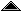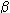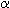..C

c
The name used in Factasia for pure combinatory logic.
calculus ratiocinator
(after Leibniz) a mechanical method of solving problems which have been expressed in a universal language known as a characteristica universalis).
casuist
a person who resolves problems of conscience or duty
cardinal
chief, fundamental
cardinal number
1. numbers denoting quantity ("one", "two", "three", ...), as opposed to ordinal numbers indicating position ("first", "second", "third", ...).

2. An equivalence class generated by the relation "same size as" obtaining when there is a one-one mapping between the elements of two sets.

3. The smallest ordinal number of some size.
large cardinal
category
a class or division
(philosophy, metaphysics)
one of the fundamental kinds of things (see: Aristotle's Categories)
(mathematics)
a kind of mathematical structure, providing in some respects a very general mathematical counterpart to the notion of a concept. A collection of objects and of morphisms (or arrows) such that each morphism has a domain and codomain which are objects, each object has an identity morphism, and morphisms compose associatively. e.g. corresponding to the mathematical concept of a group there is a category of groups which contains as objects all the groups, and as arrows between these objects the group homomorphisms.
category mistake
a favourite kind of "philosophical puzzlement" to which the Oxford philosopher Gilbert Ryle drew attention. A category mistake occurs when a speaker or writer applies a concept outside the domain in which it can meaningfully be applied (often in the course of formulating some philosophical theory).
kind
sort
type
characteristica universalis
(after Leibniz) a universal language into which any kind of problem can be translated (and then solved by calculation using a calculus ratiocinator). See: The Method of Mathematics.
class
a collection of persons or things
(logic)
sometimes used interchangeably with or instead of set, sometimes used (e.g. in NBG) for collections which are "too large" to be sets.
coerce
persuade or restrain (an unwilling person) by force
coercion (1)
the act or process of coercing
coercion (2) (computing)
the automatic conversion of a value in a computer program from one type to another as needed for the use made of the value in some particular context, e.g. the conversion of an integer to a floating point number before adding it to some other floating point number.
anarchism
cofinal
a function into an ordinal is cofinal if its range is unbounded in its codomain.
cofinality
the cofinality of an ordinal, cf(), is the least ordinalwhich maps cofinally into.
cognition
knowing, perceiving or conceiving as distinct from emotion or volition.
combinator
a function primitive to or definable in pure combinatory logic
combinatory logic
a form of logic in which bound variables are not used
complex
Not simple. Not atomic. Structured.
complex number
A number formed of two parts, so called real and imaginary parts, both of which are real numbers.
computer
An electronic device which stores and processes data following instructions which are also stored in its memory (and can therefore easily be changed).
computer algebra
the use of computer programs which automate algebraic transformations, e.g. MACSYMA, Maple, Mathematica.
conjunct
One of the immediate constituent sentences of a conjunction, e.g. in "A and B" the conjuncts are "A" and "B".
conjunction
A compound sentence of the form "A and B".
constructive
constructive logic
A logic is constructive if existence proofs in the logic depend upon constructing something with the required property and may not proceed by reductio-absurdum.
contingent
Might have been otherwise.©created 1994-9-22 modified 2004-5-2